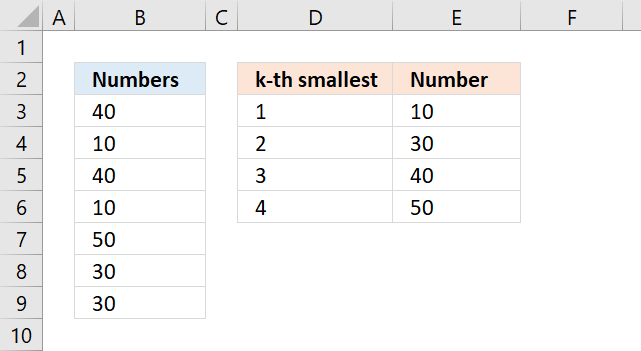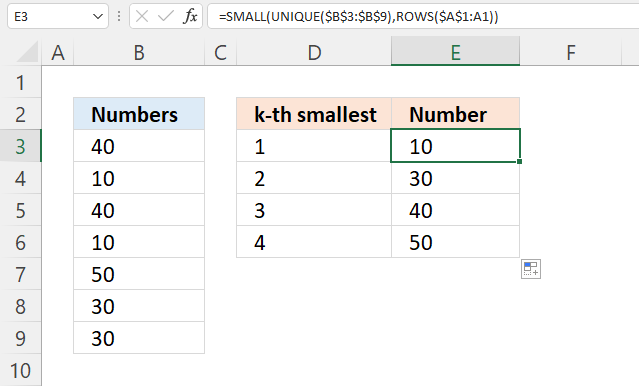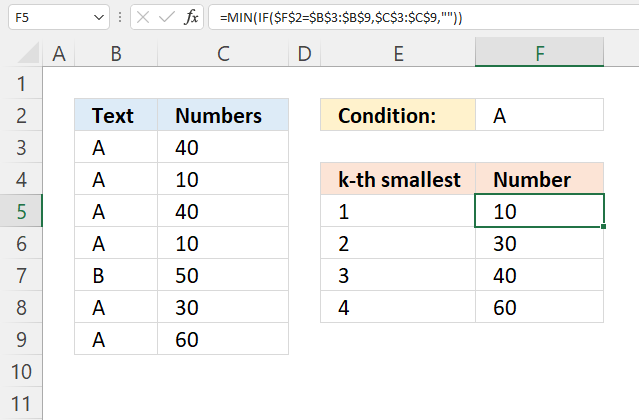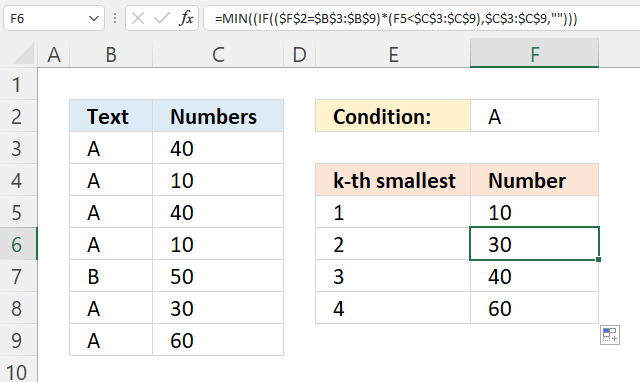Author: Oscar Cronquist Article last updated on July 09, 2022This article demonstrates ways to sort numbers from smallest to largest ignoring duplicate numbers.

## 1. SMALL function with duplicates (Excel 2016)The formulas in column E, shown in the picture above, extract the k-th smallest value from B3:B9 ignoring the duplicate numbers.

The following formula in cell E3 extracts the smallest number:

=MIN(B3:B9)

However, we need to use another formula in the cells below to ignore duplicate values. The formula in cell E4 extracts the second smallest number from B3:B9.

When you copy this formula and paste it to cells below it will extract the third, fourth, and so on, smallest value ignoring duplicate values.

=MINIFS(\$B\$3:\$B\$9, \$B\$3:\$B\$9, ">"&E3)

The MINIFS function returns the smallest value depending on the condition, in this case, it looks for values larger than the previous value in the cell above, meaning it will ignore duplicate numbers.

I can't use this formula in cell E3 because there is no formula above it.

The MINIFS function was introduced in Excel 2016, if you have an earlier version of Excel see section 2 below.

### 1.1 Explaining formula

#### Step 1 - MINIFS function

The MINIFS function calculates the smallest value based on a given set of criteria.

MINIFS(min_rangecriteria_range1criteria1, [criteria_range2criteria2], ...)

 min_range Required. A cell reference pointing to the numbers. criteria_range1 Required. Cells to evaluate based on the criteria. criteria1 Required. Criteria in the form of a number, expression, or text. [criteria_range2] Optional. Up to 126 additional arguments. [criteria2] Optional. Up to 126 additional arguments.

#### Step 2 - Populate arguments

min_range - \$B\$3:\$B\$9

criteria_range1 - \$B\$3:\$B\$9

criteria1 - ">"&E3

MINIFS(\$B\$3:\$B\$9, \$B\$3:\$B\$9, ">"&E3)

#### Step 3 - Evaluate formula

MINIFS(\$B\$3:\$B\$9, \$B\$3:\$B\$9, ">"&E3)

becomes

MINIFS({40; 10; 40; 10; 50; 30; 30},{40; 10; 40; 10; 50; 30; 30},">"&10)

and returns 30 in cell E4.Note, it won't return duplicate numbers when you copy cell E4 and paste it to the cells below.

## 2. SMALL function with duplicates (previous Excel versions)Formula in cell E3:

=MIN(B3:B9)

Array formula in cell E4:

=MIN(IF(E3<\$B\$3:\$B\$9, \$B\$3:\$B\$9, ""))

The formula above is an array formula.

### 2.1 How to enter an array formula

To enter an array formula, type the formula in a cell then press and hold CTRL + SHIFT simultaneously, now press Enter once. Release all keys.

The formula bar now shows the formula enclosed with curly brackets telling you that you entered the formula successfully. Don't enter the curly brackets yourself.

### Explaining array formula

#### Step 1 - Check which numbers are larger than cell E3

The less than sign is a logical operator, it allows you to compare numbers and text strings. The result is either TRUE or FALSE, they are boolean values and will be used in step 2 in an IF functions logical test argument.

E3<\$B\$3:\$B\$9

becomes

10<{40; 10; 40; 10; 50; 30; 30}

and returns {TRUE; FALSE; TRUE; FALSE; TRUE; TRUE; TRUE}.

#### Step 2 - Filter numbers based on logical test

The IF function returns one value if the logical test is TRUE and another value if the logical test is FALSE.

IF(logical_test, [value_if_true], [value_if_false])

IF(E3<\$B\$3:\$B\$9, \$B\$3:\$B\$9, "")

becomes

IF({TRUE; FALSE; TRUE; FALSE; TRUE; TRUE; TRUE}, \$B\$3:\$B\$9, "")

becomes

IF({TRUE; FALSE; TRUE; FALSE; TRUE; TRUE; TRUE}, {40; 10; 40; 10; 50; 30; 30}, "")

and returns {40; ""; 40; ""; 50; 30; 30}

Numbers smaller than or equal to the condition are filtered out from the array.

#### Step 3 - Extract the smallest number from the array

The MIN function returns the smallest number from a cell range or array ignoring text and blank values.

MIN(IF(E3<\$B\$3:\$B\$9, \$B\$3:\$B\$9, ""))

becomes

MIN({40; ""; 40; ""; 50; 30; 30})

and returns 30 in cell E3.

## 3. SMALL function with duplicates (Excel 365)Formula in cell E3:

=SMALL(UNIQUE(B3:B9),ROWS(\$A\$1:A1))

### 3.1 Explaining formula

#### Step 1 - Extract unique distinct values

The UNIQUE function returns unique distinct numbers.

UNIQUE(B3:B9)

becomes

UNIQUE({40; 10; 40; 10; 50; 30; 30})

and returns {40; 10; 50; 30}.

#### Step 2 - Sort values from small to large

The SMALL function returns the k-th smallest number.

SMALL(array, k)

SMALL(UNIQUE(B3:B9),ROWS(\$A\$1:A1))

becomes

SMALL({40; 10; 50; 30},ROWS(\$A\$1:A1))

The ROWS function returns the number of rows based on the specified cell reference.

ROWS(ref)

The reference \$A\$1:A1 contains an absolute part and a relative part, the absolute part stays the same, however, the relative part changes when you copy the cell and paste it to cells below.

SMALL({40; 10; 50; 30},ROWS(\$A\$1:A1))

becomes

SMALL({40; 10; 50; 30},1)

and returns 10 in cell E3.

## 4. SMALL function ignore duplicates based on a conditionThe image above demonstrates two formulas that let you extract the smallest number based on a condition ignoring duplicate numbers.

The formula in cell F5 extracts the smallest number based on a condition specified in cell F2, however, this formula works only in the first cell. Cell F6 and cells below require a different formula.

The array formulas below work in all Excel versions, here is how to enter an array formula.

Array formula in cell F5:

=MIN(IF(\$F\$2=\$B\$3:\$B\$9, \$C\$3:\$C\$9, ""))

Array formula in cell F6:

=MIN(IF((\$F\$2=\$B\$3:\$B\$9)*(F5<\$C\$3:\$C\$9),\$C\$3:\$C\$9,""))

Copy cell F6 and paste it to cells below as far as needed.

### 4.1 Explaining formula in cell F5

#### Step 1 - Logical test

The equal sign is a logical operator and the result is a boolean value, TRUE or FALSE.

\$F\$2=\$B\$3:\$B\$9

becomes

"A"={"A"; "A"; "A"; "A"; "B"; "A"; "A"}

and returns {TRUE; TRUE; TRUE; TRUE; FALSE; TRUE; TRUE}.

#### Step 2 - Evaluate IF function

The IF function returns one value if the logical test is TRUE and another value if the logical test is FALSE.

IF(logical_test, [value_if_true], [value_if_false])

IF(\$F\$2=\$B\$3:\$B\$9, \$C\$3:\$C\$9, "")

becomes

IF({TRUE; TRUE; TRUE; TRUE; FALSE; TRUE; TRUE}, \$C\$3:\$C\$9, "")

becomes

IF({TRUE; TRUE; TRUE; TRUE; FALSE; TRUE; TRUE}, {40; 10; 40; 10; 50; 30; 60}, "")

and returns {40; 10; 40; 10; ""; 30; 60}.

#### Step 3 - Return smallest number

The MIN function returns the smallest number from a cell range or array ignoring text and blank values.

MIN(cell_ref)

MIN(IF(\$F\$2=\$B\$3:\$B\$9, \$C\$3:\$C\$9, ""))

becomes

MIN({40; 10; 40; 10; ""; 30; 60})

and returns 10.

### 4.2 Explaining formula in cell F6#### Step 1 - Identify values equal to condition

The equal sign is a logical operator and the result is a boolean value, TRUE or FALSE.

\$F\$2=\$B\$3:\$B\$9

becomes

"A"={"A"; "A"; "A"; "A"; "B"; "A"; "A"}

and returns {TRUE; TRUE; TRUE; TRUE; FALSE; TRUE; TRUE}.

#### Step 2 - Check if numbers are smaller than previous number above

The less than sign is a logical operator, it allows you to compare numbers and text strings. The result is either TRUE or FALSE, they are boolean values.

F5<\$C\$3:\$C\$9

becomes

10<{40; 10; 40; 10; 50; 30; 60}

and returns

{TRUE; FALSE; TRUE; FALSE; TRUE; TRUE; TRUE}

#### Step 3 - Multiply arrays

Both test must be true and to do that we need to multiply the array, in other words, apply AND logic.

Use the asterisk to multiply values or arrays.

* (asterisk) - Both logical expressions must match (AND logic)

The AND logic behind this is that

• TRUE * TRUE = TRUE (1)
• TRUE * FALSE = FALSE (0)
• FALSE * FALSE = FALSE (0)

(\$F\$2=\$B\$3:\$B\$9)*(F5<\$C\$3:\$C\$9)

The parentheses control the order of operation.

(\$F\$2=\$B\$3:\$B\$9)*(F5<\$C\$3:\$C\$9)

becomes

{TRUE; TRUE; TRUE; TRUE; FALSE; TRUE; TRUE}*{TRUE; FALSE; TRUE; FALSE; TRUE; TRUE; TRUE}

and returns {1; 0; 1; 0; 0; 1; 1}.

#### Step 4 - Replace 1 with corresponding number

The IF function returns one value if the logical test is TRUE and another value if the logical test is FALSE.

IF(logical_test, [value_if_true], [value_if_false])

IF((\$F\$2=\$B\$3:\$B\$9)*(F5<\$C\$3:\$C\$9),\$C\$3:\$C\$9,"")

becomes

IF({1; 0; 1; 0; 0; 1; 1},\$C\$3:\$C\$9,"")

becomes

IF({1; 0; 1; 0; 0; 1; 1},{40; 10; 40; 10; 50; 30; 60},"")

and returns {40; ""; 40; ""; ""; 30; 60}.

#### Step 5 - Extract the smallest number from the array

The MIN function returns the smallest number from a cell range or array ignoring text and blank values.

MIN(cell_ref)

MIN(IF((\$F\$2=\$B\$3:\$B\$9)*(F5<\$C\$3:\$C\$9),\$C\$3:\$C\$9,"")))

becomes

MIN({40; ""; 40; ""; ""; 30; 60})

and returns 30.